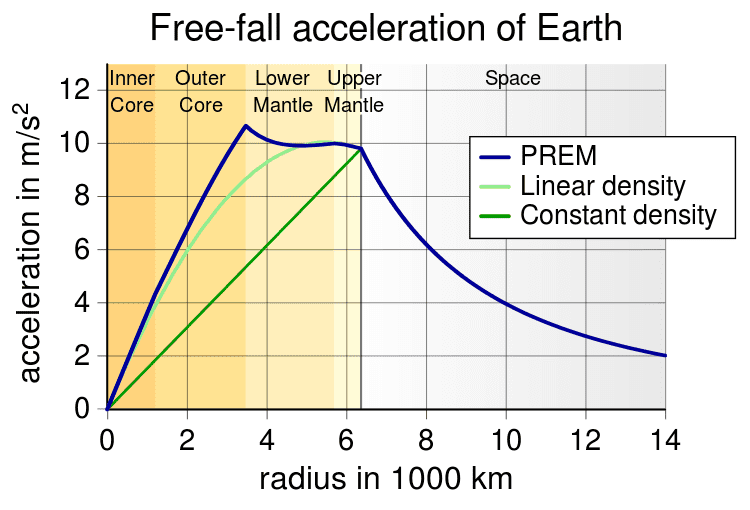• B
• Lim Y K

#### Lim Y K

When a stone is place on TOP of a very tall mountain, does the gravitational force acting on it decreases? And if a stone is on the ground at sea level, is the gravitational force acting on the stone the highest?

If you drop the rock from an airplane, is the gravitational force acting on it the highest?

Is centre of gravity a force?

When a stone is place on TOP of a very tall mountain, does the gravitational force acting on it decreases? And if a stone is on the ground at sea level, is the gravitational force acting on the stone the highest?

Yes, that's pretty much correct. Gravitational force decreases with height, so, in general, the further above the ground you are, the lower the force of gravity is.

If you drop the rock from an airplane, is the gravitational force acting on it the highest?

No. The force of gravity would increase as the stone falls.

Is centre of gravity a force?

It is not. The center of gravity is very similar to the center of mass of an object, but I think there may be some slight differences between the two.

The center of gravity is very similar to the center of mass of an object, but I think there may be some slight differences between the two.

Consider a uniform rod. Its center of mass is at the midpoint.

In a uniform gravitational field, its center of gravity is also at the midpoint: you can balance the rod horizontally on your finger at its midpoint.

Now suppose the gravitational field is not uniform, but instead is stronger towards the right end of the bar than the left end, so the right end of the bar is pulled down more strongly than the left end. In order to balance the rod, you have to put your finger closer to the right end: your finger's position is the center of gravity in this case.

In practice, the difference between the center of mass and the center of gravity is significant only when the object is large enough for either the magnitude or direction of the gravitational field to vary significantly for different parts of the object.

Last edited:
•Drakkith
The force of gravity can be found using the equation

Fg = G(m1m2)/r2

R refers to the distance between the centers of the two bodies so as the distance increases this leads to the force of gravity decreasing

On Earth for humans this potential change is not very noticible even if you were to go to the top of Mount Everest but for the force of gravity between the Earth and a satellite orbiting Earth the change in the force of gravity is obviously significant

The rest was pretty much covered by Drakkith and jtbell

Last edited by a moderator:
The force of gravity can be found using the equation

Fg = G(m1+m2)/r2

R refers to the distance between the centers of the two bodies so as the distance increases this leads to the force of gravity decreasing

On Earth for humans this potential change is not very noticible even if you were to go to the top of Mount Everest but for the force of gravity between the Earth and a satellite orbiting Earth the change in the force of gravity is obviously significant

The rest was pretty much covered by Drakkith and jtbell
No, it's not the sum of the masses but their product.

No, it's not the sum of the masses but their product.

That's what I get for relying on memory

Thanks for pointing it out

I will edit my post accordingly

A couple of points. The formula as given is correct, though it does not accurately reflect the makeup of the Earth. As you approach the surface of a a sphere the force of gravity goes up by a inverse radius squared factor. For a uniform sphere that force decreases linearly as one go from the surface to the center. The problem is that that formula assumes a uniform density and the Earth is far from uniform. The core is much denser than the mantle. As a result as one goes deeper into the Earth the force of gravity would increase until one hit the outer core mantle boundary, at which point it would begin to decrease:By Con-struct - Dziewonski, A.M. (1981). "Preliminary reference Earth model". Physics of the Earth and Planetary Interiors 25: 297–356.http://geophysics.ou.edu/solid_earth/prem.htmlAllenMcC [Broken]., File:EarthGravityPREM.jpg for the linear density curve, CC BY-SA 3.0, https://commons.wikimedia.org/w/index.php?curid=27390559

The straight line shows gravity inside the Earth for a constant density, the curved blue line is based upon our current knowledge of the interior density of the Earth.

Last edited by a moderator:
The formula as given is correct, though it does not accurately reflect the makeup of the Earth.

It's not supposed to. That formula, as written above, is only valid when you're outside of the Earth. And even then it isn't 100% accurate since the Earth is not a perfect sphere.